Sound

# Speed of Sound

### Learning Objectives

By the end of this section, you will be able to:

• Explain the relationship between wavelength and frequency of sound
• Determine the speed of sound in different media
• Derive the equation for the speed of sound in air
• Determine the speed of sound in air for a given temperature

Sound, like all waves, travels at a certain speed and has the properties of frequency and wavelength. You can observe direct evidence of the speed of sound while watching a fireworks display ((Figure)). You see the flash of an explosion well before you hear its sound and possibly feel the pressure wave, implying both that sound travels at a finite speed and that it is much slower than light.

When a firework shell explodes, we perceive the light energy before the sound energy because sound travels more slowly than light does.The difference between the speed of light and the speed of sound can also be experienced during an electrical storm. The flash of lighting is often seen before the clap of thunder. You may have heard that if you count the number of seconds between the flash and the sound, you can estimate the distance to the source. Every five seconds converts to about one mile. The velocity of any wave is related to its frequency and wavelength by

$v=f\lambda ,$

where v is the speed of the wave, f is its frequency, and $\lambda$ is its wavelength. Recall from Waves that the wavelength is the length of the wave as measured between sequential identical points. For example, for a surface water wave or sinusoidal wave on a string, the wavelength can be measured between any two convenient sequential points with the same height and slope, such as between two sequential crests or two sequential troughs. Similarly, the wavelength of a sound wave is the distance between sequential identical parts of a wave—for example, between sequential compressions ((Figure)). The frequency is the same as that of the source and is the number of waves that pass a point per unit time.

A sound wave emanates from a source, such as a tuning fork, vibrating at a frequency f. It propagates at speed v and has a wavelength $\lambda$.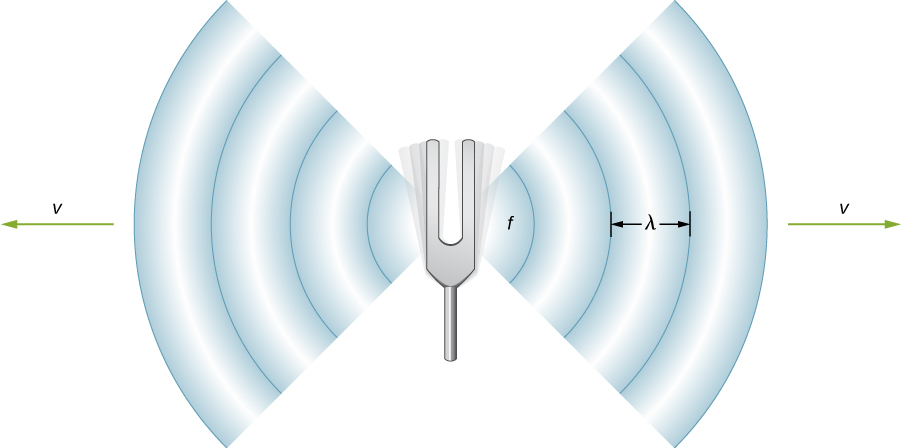### Speed of Sound in Various Media

(Figure) shows that the speed of sound varies greatly in different media. The speed of sound in a medium depends on how quickly vibrational energy can be transferred through the medium. For this reason, the derivation of the speed of sound in a medium depends on the medium and on the state of the medium. In general, the equation for the speed of a mechanical wave in a medium depends on the square root of the restoring force, or the elastic property, divided by the inertial property,

$v=\sqrt{\frac{\text{elastic}\phantom{\rule{0.2em}{0ex}}\text{property}}{\text{inertial}\phantom{\rule{0.2em}{0ex}}\text{property}}}.$

Also, sound waves satisfy the wave equation derived in Waves,

$\frac{{\partial }^{2}y\left(x,t\right)}{\partial {x}^{2}}=\frac{1}{{v}^{2}}\phantom{\rule{0.2em}{0ex}}\frac{{\partial }^{2}y\left(x,t\right)}{\partial {t}^{2}}.$

Recall from Waves that the speed of a wave on a string is equal to $v=\sqrt{\frac{{F}_{T}}{\mu }},$ where the restoring force is the tension in the string ${F}_{T}$ and the linear density $\mu$ is the inertial property. In a fluid, the speed of sound depends on the bulk modulus and the density,

$v=\sqrt{\frac{\beta }{\rho }}.$

The speed of sound in a solid the depends on the Young’s modulus of the medium and the density,

$v=\sqrt{\frac{Y}{\rho }}.$

In an ideal gas (see The Kinetic Theory of Gases), the equation for the speed of sound is

$v=\sqrt{\frac{\gamma R{T}_{\text{K}}}{M}},$

where $\gamma$ is the adiabatic index, $R=8.31\phantom{\rule{0.2em}{0ex}}\text{J/mol}·\text{K}$ is the gas constant, ${T}_{\text{K}}$ is the absolute temperature in kelvins, and M is the molecular mass. In general, the more rigid (or less compressible) the medium, the faster the speed of sound. This observation is analogous to the fact that the frequency of simple harmonic motion is directly proportional to the stiffness of the oscillating object as measured by k, the spring constant. The greater the density of a medium, the slower the speed of sound. This observation is analogous to the fact that the frequency of a simple harmonic motion is inversely proportional to m, the mass of the oscillating object. The speed of sound in air is low, because air is easily compressible. Because liquids and solids are relatively rigid and very difficult to compress, the speed of sound in such media is generally greater than in gases.

Speed of Sound in Various Media
Medium v (m/s)
Gases at$0\text{°}C$
Air 331
Carbon dioxide 259
Oxygen 316
Helium 965
Hydrogen 1290
Liquids at$20\text{°}C$
Ethanol 1160
Mercury 1450
Water, fresh 1480
Sea Water 1540
Human tissue 1540
Solids (longitudinal or bulk)
Vulcanized rubber 54
Polyethylene 920
Marble 3810
Glass, Pyrex 5640
Aluminum 5120
Steel 5960

Because the speed of sound depends on the density of the material, and the density depends on the temperature, there is a relationship between the temperature in a given medium and the speed of sound in the medium. For air at sea level, the speed of sound is given by

$v=\phantom{\rule{0.2em}{0ex}}331\frac{\text{m}}{\text{s}}\sqrt{1+\frac{{T}_{\text{C}}}{273\text{°}\text{C}}}=331\frac{\text{m}}{\text{s}}\sqrt{\frac{{T}_{\text{K}}}{273\phantom{\rule{0.2em}{0ex}}\text{K}}}$

where the temperature in the first equation (denoted as ${T}_{\text{C}}$) is in degrees Celsius and the temperature in the second equation (denoted as ${T}_{\text{K}}$) is in kelvins. The speed of sound in gases is related to the average speed of particles in the gas, ${v}_{\text{rms}}=\sqrt{\frac{3{k}_{\text{B}}T}{m}},$ where ${k}_{\text{B}}$ is the Boltzmann constant $\left(1.38\phantom{\rule{0.2em}{0ex}}×\phantom{\rule{0.2em}{0ex}}{10}^{-23}\phantom{\rule{0.2em}{0ex}}\text{J/K}\right)$ and m is the mass of each (identical) particle in the gas. Note that v refers to the speed of the coherent propagation of a disturbance (the wave), whereas ${v}_{\text{rms}}$ describes the speeds of particles in random directions. Thus, it is reasonable that the speed of sound in air and other gases should depend on the square root of temperature. While not negligible, this is not a strong dependence. At $0\text{°C}$, the speed of sound is 331 m/s, whereas at $20.0\text{°C}$, it is 343 m/s, less than a $4\text{%}$ increase. (Figure) shows how a bat uses the speed of sound to sense distances.

A bat uses sound echoes to find its way about and to catch prey. The time for the echo to return is directly proportional to the distance.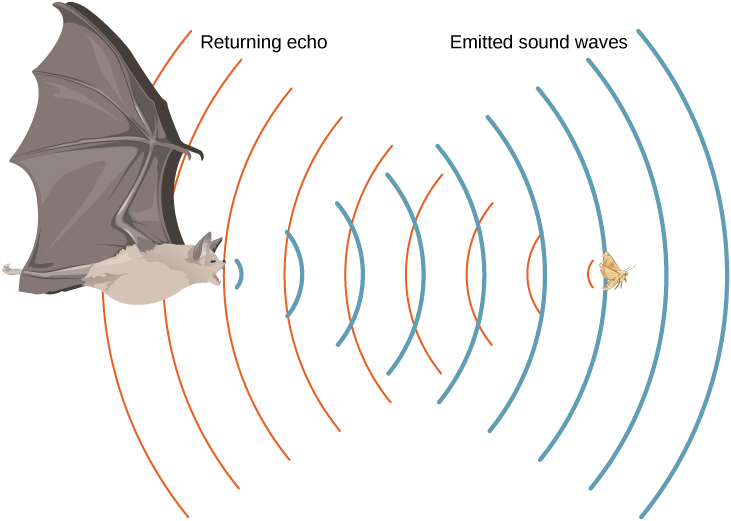### Derivation of the Speed of Sound in Air

As stated earlier, the speed of sound in a medium depends on the medium and the state of the medium. The derivation of the equation for the speed of sound in air starts with the mass flow rate and continuity equation discussed in Fluid Mechanics.

Consider fluid flow through a pipe with cross-sectional area A ((Figure)). The mass in a small volume of length x of the pipe is equal to the density times the volume, or $m=\rho V=\rho Ax.$ The mass flow rate is

$\frac{dm}{dt}=\frac{d}{dt}\left(\rho V\right)=\frac{d}{dt}\left(\rho Ax\right)=\rho A\frac{dx}{dt}=\rho Av.$

The continuity equation from Fluid Mechanics states that the mass flow rate into a volume has to equal the mass flow rate out of the volume, ${\rho }_{\text{in}}{A}_{\text{in}}{v}_{\text{in}}={\rho }_{\text{out}}{A}_{\text{out}}{v}_{\text{out}}.$

The mass of a fluid in a volume is equal to the density times the volume, $m=\rho V=\rho Ax.$ The mass flow rate is the time derivative of the mass.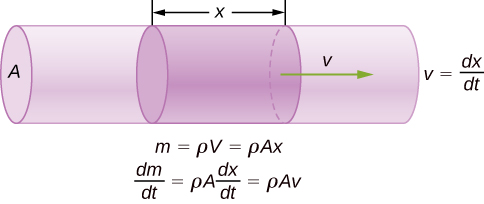Now consider a sound wave moving through a parcel of air. A parcel of air is a small volume of air with imaginary boundaries ((Figure)). The density, temperature, and velocity on one side of the volume of the fluid are given as $\rho ,T,v,$ and on the other side are $\rho +d\rho ,T+dT,v+dv.$

A sound wave moves through a volume of fluid. The density, temperature, and velocity of the fluid change from one side to the other.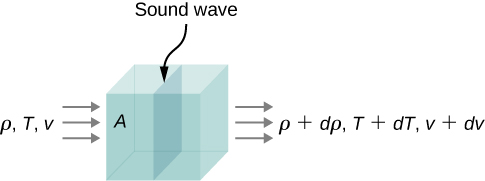The continuity equation states that the mass flow rate entering the volume is equal to the mass flow rate leaving the volume, so

$\rho Av=\left(\rho +d\rho \right)A\left(v+dv\right).$

This equation can be simplified, noting that the area cancels and considering that the multiplication of two infinitesimals is approximately equal to zero: $d\rho \left(dv\right)\approx 0,$

$\begin{array}{ccc}\hfill \rho v& =\hfill & \left(\rho +d\rho \right)\left(v+dv\right)\hfill \\ \hfill \rho v& =\hfill & \rho v+\rho \left(dv\right)+\left(d\rho \right)v+\left(d\rho \right)\left(dv\right)\hfill \\ \hfill 0& =\hfill & \rho \left(dv\right)+\left(d\rho \right)v\hfill \\ \hfill \rho \phantom{\rule{0.2em}{0ex}}dv& =\hfill & \text{−}vd\rho .\hfill \end{array}$

The net force on the volume of fluid ((Figure)) equals the sum of the forces on the left face and the right face:

$\begin{array}{ccc}\hfill {F}_{\text{net}}& =\hfill & p\phantom{\rule{0.2em}{0ex}}dy\phantom{\rule{0.2em}{0ex}}dz-\left(p+dp\right)dy\phantom{\rule{0.2em}{0ex}}dz\hfill \\ & =\hfill & p\phantom{\rule{0.2em}{0ex}}dy\phantom{\rule{0.2em}{0ex}}dz-pdy\phantom{\rule{0.2em}{0ex}}dz-dp\phantom{\rule{0.2em}{0ex}}dy\phantom{\rule{0.2em}{0ex}}dz\hfill \\ & =\hfill & \text{−}dp\phantom{\rule{0.2em}{0ex}}dy\phantom{\rule{0.2em}{0ex}}dz\hfill \\ \hfill ma& =\hfill & \text{−}dp\phantom{\rule{0.2em}{0ex}}dy\phantom{\rule{0.2em}{0ex}}dz.\hfill \end{array}$
A sound wave moves through a volume of fluid. The force on each face can be found by the pressure times the area.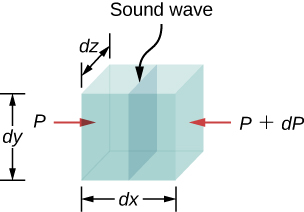The acceleration is the force divided by the mass and the mass is equal to the density times the volume, $m=\rho V=\rho \phantom{\rule{0.2em}{0ex}}dx\phantom{\rule{0.2em}{0ex}}dy\phantom{\rule{0.2em}{0ex}}dz.$ We have

$\begin{array}{ccc}\hfill ma& =\hfill & \text{−}dp\phantom{\rule{0.2em}{0ex}}dy\phantom{\rule{0.2em}{0ex}}dz\hfill \\ \hfill a& =\hfill & -\frac{dp\phantom{\rule{0.2em}{0ex}}dy\phantom{\rule{0.2em}{0ex}}dz}{m}=-\frac{dp\phantom{\rule{0.2em}{0ex}}dy\phantom{\rule{0.2em}{0ex}}dz}{\rho \phantom{\rule{0.2em}{0ex}}dx\phantom{\rule{0.2em}{0ex}}dy\phantom{\rule{0.2em}{0ex}}dz}=-\frac{dp}{\left(\rho \phantom{\rule{0.2em}{0ex}}dx\right)}\hfill \\ \hfill \frac{dv}{dt}& =\hfill & -\frac{dp}{\left(\rho \phantom{\rule{0.2em}{0ex}}dx\right)}\hfill \\ \hfill dv& =\hfill & -\frac{dp}{\left(\rho \phantom{\rule{0.2em}{0ex}}dx\right)}dt=-\frac{dp}{\rho }\phantom{\rule{0.2em}{0ex}}\frac{1}{v}\hfill \\ \hfill \rho v\phantom{\rule{0.2em}{0ex}}dv& =\hfill & \text{−}dp.\hfill \end{array}$

From the continuity equation $\rho \phantom{\rule{0.2em}{0ex}}dv=\text{−}vd\rho$, we obtain

$\begin{array}{ccc}\hfill \rho vdv& =\hfill & \text{−}dp\hfill \\ \hfill \left(\text{−}vd\rho \right)v& =\hfill & \text{−}dp\hfill \\ \hfill v& =\hfill & \sqrt{\frac{dp}{d\rho }}.\hfill \end{array}$

Consider a sound wave moving through air. During the process of compression and expansion of the gas, no heat is added or removed from the system. A process where heat is not added or removed from the system is known as an adiabatic system. Adiabatic processes are covered in detail in The First Law of Thermodynamics, but for now it is sufficient to say that for an adiabatic process,$p{V}^{\gamma }=\text{constant,}$ where p is the pressure, V is the volume, and gamma $\left(\gamma \right)$ is a constant that depends on the gas. For air, $\gamma =1.40$. The density equals the number of moles times the molar mass divided by the volume, so the volume is equal to $V=\frac{nM}{\rho }.$ The number of moles and the molar mass are constant and can be absorbed into the constant $p{\left(\frac{1}{\rho }\right)}^{\gamma }=\text{constant}\text{.}$ Taking the natural logarithm of both sides yields $\text{ln}\phantom{\rule{0.2em}{0ex}}p-\gamma \phantom{\rule{0.2em}{0ex}}\text{ln}\phantom{\rule{0.2em}{0ex}}\rho =\text{constant}\text{.}$ Differentiating with respect to the density, the equation becomes

$\begin{array}{ccc}\hfill \text{ln}\phantom{\rule{0.2em}{0ex}}p-\gamma \phantom{\rule{0.2em}{0ex}}\text{ln}\phantom{\rule{0.2em}{0ex}}\rho & =\hfill & \text{constant}\hfill \\ \hfill \frac{d}{d\rho }\left(\text{ln}\phantom{\rule{0.2em}{0ex}}p-\gamma \phantom{\rule{0.2em}{0ex}}\text{ln}\phantom{\rule{0.2em}{0ex}}\rho \right)& =\hfill & \frac{d}{d\rho }\left(\text{constant}\right)\hfill \\ \hfill \frac{1}{p}\phantom{\rule{0.2em}{0ex}}\frac{dp}{d\rho }-\frac{\gamma }{\rho }& =\hfill & 0\hfill \\ \hfill \frac{dp}{d\rho }& =\hfill & \frac{\gamma p}{\rho }.\hfill \end{array}$

If the air can be considered an ideal gas, we can use the ideal gas law:

$\begin{array}{ccc}\hfill pV& =\hfill & nRT=\frac{m}{M}RT\hfill \\ \hfill p& =\hfill & \frac{m}{V}\phantom{\rule{0.2em}{0ex}}\frac{RT}{M}=\rho \frac{RT}{M}.\hfill \end{array}$

Here M is the molar mass of air:

$\frac{dp}{d\rho }=\frac{\gamma p}{\rho }=\frac{\gamma \left(\rho \frac{RT}{M}\right)}{\rho }=\frac{\gamma RT}{M}.$

Since the speed of sound is equal to $v=\sqrt{\frac{dp}{d\rho }}$, the speed is equal to

$v=\sqrt{\frac{\gamma \phantom{\rule{0.2em}{0ex}}RT}{M}}.$

Note that the velocity is faster at higher temperatures and slower for heavier gases. For air, $\gamma =1.4,$ $M=0.02897\frac{\text{kg}}{\text{mol}},$ and $R=8.31\frac{\text{J}}{\text{mol}·\text{K}}.$ If the temperature is ${T}_{\text{C}}=20\text{°}\text{C}\left(T=293\phantom{\rule{0.2em}{0ex}}\text{K}\right),$ the speed of sound is $v=343\phantom{\rule{0.2em}{0ex}}\text{m/s}\text{.}$

The equation for the speed of sound in air $v=\sqrt{\frac{\gamma RT}{M}}$ can be simplified to give the equation for the speed of sound in air as a function of absolute temperature:

$\begin{array}{cc}\hfill v& =\sqrt{\frac{\gamma RT}{M}}\hfill \\ & =\sqrt{\frac{\gamma RT}{M}\left(\frac{273\phantom{\rule{0.2em}{0ex}}\text{K}}{273\phantom{\rule{0.2em}{0ex}}\text{K}}\right)}=\sqrt{\frac{\left(273\phantom{\rule{0.2em}{0ex}}\text{K}\right)\gamma R}{M}}\sqrt{\frac{T}{273\phantom{\rule{0.2em}{0ex}}\text{K}}}\hfill \\ & \approx 331\frac{\text{m}}{\text{s}}\sqrt{\frac{T}{273\phantom{\rule{0.2em}{0ex}}\text{K}}.}\hfill \end{array}$

One of the more important properties of sound is that its speed is nearly independent of the frequency. This independence is certainly true in open air for sounds in the audible range. If this independence were not true, you would certainly notice it for music played by a marching band in a football stadium, for example. Suppose that high-frequency sounds traveled faster—then the farther you were from the band, the more the sound from the low-pitch instruments would lag that from the high-pitch ones. But the music from all instruments arrives in cadence independent of distance, so all frequencies must travel at nearly the same speed. Recall that

$v=f\lambda .$

In a given medium under fixed conditions, v is constant, so there is a relationship between f and $\lambda ;$ the higher the frequency, the smaller the wavelength ((Figure)).

Because they travel at the same speed in a given medium, low-frequency sounds must have a greater wavelength than high-frequency sounds. Here, the lower-frequency sounds are emitted by the large speaker, called a woofer, whereas the higher-frequency sounds are emitted by the small speaker, called a tweeter.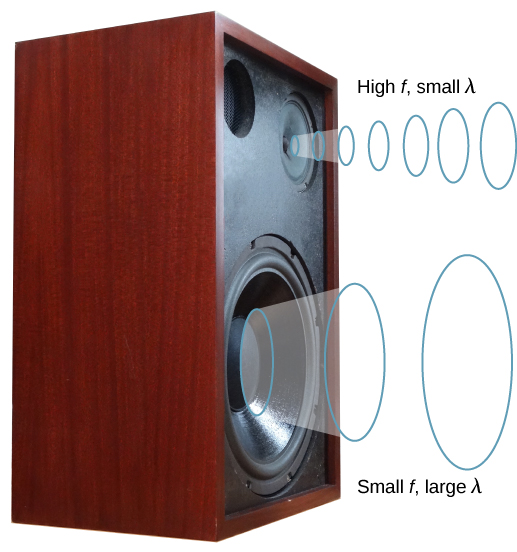Calculating Wavelengths
Calculate the wavelengths of sounds at the extremes of the audible range, 20 and 20,000 Hz, in $30.0\text{°C}$ air. (Assume that the frequency values are accurate to two significant figures.)

Strategy
To find wavelength from frequency, we can use $v=f\lambda .$

Solution

1. Identify knowns. The value for v is given by
$v=\left(331\phantom{\rule{0.2em}{0ex}}\text{m/s}\right)\sqrt{\frac{T}{273\phantom{\rule{0.2em}{0ex}}\text{K}}}.$
2. Convert the temperature into kelvins and then enter the temperature into the equation
$v=\left(331\phantom{\rule{0.2em}{0ex}}\text{m/s}\right)\sqrt{\frac{303\phantom{\rule{0.2em}{0ex}}\text{K}}{273\phantom{\rule{0.2em}{0ex}}\text{K}}}=348.7\phantom{\rule{0.2em}{0ex}}\text{m/s}\text{.}$
3. Solve the relationship between speed and wavelength for λ:
$\lambda =\frac{v}{f}.$
4. Enter the speed and the minimum frequency to give the maximum wavelength:
${\lambda }_{\text{max}}=\text{​}\frac{348.7\phantom{\rule{0.2em}{0ex}}\text{m/s}}{20\phantom{\rule{0.2em}{0ex}}\text{Hz}}=17\phantom{\rule{0.2em}{0ex}}\text{m}\text{.}$
5. Enter the speed and the maximum frequency to give the minimum wavelength:
${\lambda }_{\text{min}}=\frac{348.7\phantom{\rule{0.2em}{0ex}}\text{m/s}}{20,000\phantom{\rule{0.2em}{0ex}}\text{Hz}}=0.017\phantom{\rule{0.2em}{0ex}}\text{m}=1.7\phantom{\rule{0.2em}{0ex}}\text{cm}\text{.}$

Significance
Because the product of f multiplied by $\lambda$ equals a constant, the smaller f is, the larger $\lambda$ must be, and vice versa.

The speed of sound can change when sound travels from one medium to another, but the frequency usually remains the same. This is similar to the frequency of a wave on a string being equal to the frequency of the force oscillating the string. If v changes and f remains the same, then the wavelength $\lambda$ must change. That is, because $v=f\lambda$, the higher the speed of a sound, the greater its wavelength for a given frequency.

Check Your Understanding Imagine you observe two firework shells explode. You hear the explosion of one as soon as you see it. However, you see the other shell for several milliseconds before you hear the explosion. Explain why this is so.

Sound and light both travel at definite speeds, and the speed of sound is slower than the speed of light. The first shell is probably very close by, so the speed difference is not noticeable. The second shell is farther away, so the light arrives at your eyes noticeably sooner than the sound wave arrives at your ears.

Although sound waves in a fluid are longitudinal, sound waves in a solid travel both as longitudinal waves and transverse waves. Seismic waves, which are essentially sound waves in Earth’s crust produced by earthquakes, are an interesting example of how the speed of sound depends on the rigidity of the medium. Earthquakes produce both longitudinal and transverse waves, and these travel at different speeds. The bulk modulus of granite is greater than its shear modulus. For that reason, the speed of longitudinal or pressure waves (P-waves) in earthquakes in granite is significantly higher than the speed of transverse or shear waves (S-waves). Both types of earthquake waves travel slower in less rigid material, such as sediments. P-waves have speeds of 4 to 7 km/s, and S-waves range in speed from 2 to 5 km/s, both being faster in more rigid material. The P-wave gets progressively farther ahead of the S-wave as they travel through Earth’s crust. The time between the P- and S-waves is routinely used to determine the distance to their source, the epicenter of the earthquake. Because S-waves do not pass through the liquid core, two shadow regions are produced ((Figure)).

Earthquakes produce both longitudinal waves (P-waves) and transverse waves (S-waves), and these travel at different speeds. Both waves travel at different speeds in the different regions of Earth, but in general, P-waves travel faster than S-waves. S-waves cannot be supported by the liquid core, producing shadow regions.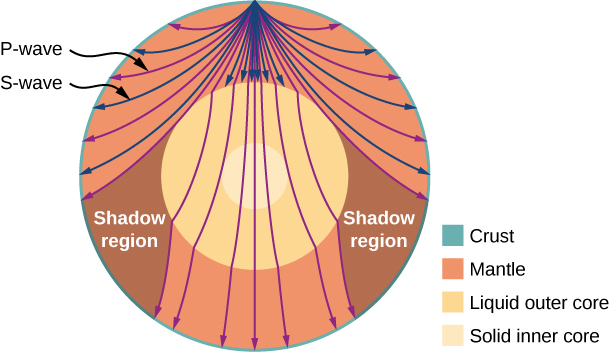As sound waves move away from a speaker, or away from the epicenter of an earthquake, their power per unit area decreases. This is why the sound is very loud near a speaker and becomes less loud as you move away from the speaker. This also explains why there can be an extreme amount of damage at the epicenter of an earthquake but only tremors are felt in areas far from the epicenter. The power per unit area is known as the intensity, and in the next section, we will discuss how the intensity depends on the distance from the source.

### Summary

• The speed of sound depends on the medium and the state of the medium.
• In a fluid, because the absence of shear forces, sound waves are longitudinal. A solid can support both longitudinal and transverse sound waves.
• In air, the speed of sound is related to air temperature T by$v=\phantom{\rule{0.2em}{0ex}}331\frac{\text{m}}{\text{s}}\sqrt{\frac{{T}_{\text{K}}}{273\phantom{\rule{0.2em}{0ex}}\text{K}}}=331\frac{\text{m}}{\text{s}}\sqrt{1+\frac{{T}_{\text{C}}}{273\text{°}\text{C}}}.$
• v is the same for all frequencies and wavelengths of sound in air.

### Conceptual Questions

How do sound vibrations of atoms differ from thermal motion?

When sound passes from one medium to another where its propagation speed is different, does its frequency or wavelength change? Explain your answer briefly.

The frequency does not change as the sound wave moves from one medium to another. Since the speed changes and the frequency does not, the wavelength must change. This is similar to the driving force of a harmonic oscillator or a wave on the string.

A popular party trick is to inhale helium and speak in a high-frequency, funny voice. Explain this phenomenon.

You may have used a sonic range finder in lab to measure the distance of an object using a clicking sound from a sound transducer. What is the principle used in this device?

The transducer sends out a sound wave, which reflects off the object in question and measures the time it takes for the sound wave to return. Since the speed of sound is constant, the distance to the object can found by multiplying the velocity of sound by half the time interval measured.

The sonic range finder discussed in the preceding question often needs to be calibrated. During the calibration, the software asks for the room temperature. Why do you suppose the room temperature is required?

### Problems

When poked by a spear, an operatic soprano lets out a 1200-Hz shriek. What is its wavelength if the speed of sound is 345 m/s?

What frequency sound has a 0.10-m wavelength when the speed of sound is 340 m/s?

$f=3400\phantom{\rule{0.2em}{0ex}}\text{Hz}$

Calculate the speed of sound on a day when a 1500-Hz frequency has a wavelength of 0.221 m.

(a) What is the speed of sound in a medium where a 100-kHz frequency produces a 5.96-cm wavelength? (b) Which substance in (Figure) is this likely to be?

a. $v=5.96\phantom{\rule{0.2em}{0ex}}×\phantom{\rule{0.2em}{0ex}}{10}^{3}\phantom{\rule{0.2em}{0ex}}\text{m/s}$; b. steel (from value in (Figure))

Show that the speed of sound in $20.0\text{°}\text{C}$ air is $343\phantom{\rule{0.2em}{0ex}}\text{m/s},$ as claimed in the text.

Air temperature in the Sahara Desert can reach $56.0\text{°}\text{C}$ (about $134\text{°}\text{F}$). What is the speed of sound in air at that temperature?

$v=363\phantom{\rule{0.2em}{0ex}}\frac{\text{m}}{\text{s}}$

Dolphins make sounds in air and water. What is the ratio of the wavelength of a sound in air to its wavelength in seawater? Assume air temperature is $20.0\text{°}\text{C}\text{.}$

A sonar echo returns to a submarine 1.20 s after being emitted. What is the distance to the object creating the echo? (Assume that the submarine is in the ocean, not in fresh water.)

$\text{Δ}x=924\phantom{\rule{0.2em}{0ex}}\text{m}$

(a) If a submarine’s sonar can measure echo times with a precision of 0.0100 s, what is the smallest difference in distances it can detect? (Assume that the submarine is in the ocean, not in fresh water.) (b) Discuss the limits this time resolution imposes on the ability of the sonar system to detect the size and shape of the object creating the echo.

Ultrasonic sound waves are often used in methods of nondestructive testing. For example, this method can be used to find structural faults in a steel I-beams used in building. Consider a 10.00 meter long, steel I-beam with a cross-section shown below. The weight of the I-beam is 3846.50 N. What would be the speed of sound through in the I-beam? $\left({Y}_{\text{steel}}=200\phantom{\rule{0.2em}{0ex}}\text{GPa},{\beta }_{\text{steel}}=159\phantom{\rule{0.2em}{0ex}}\text{GPa}\right)$.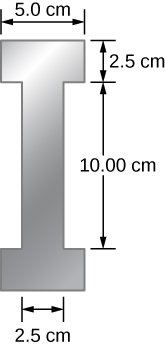$\begin{array}{}\\ \\ V=0.05\phantom{\rule{0.2em}{0ex}}{\text{m}}^{3}\hfill \\ m=392.5\phantom{\rule{0.2em}{0ex}}\text{kg}\hfill \\ \rho =7850\phantom{\rule{0.2em}{0ex}}{\text{kg/m}}^{3}\hfill \\ v=5047.54\phantom{\rule{0.2em}{0ex}}\text{m/s}\hfill \end{array}$

A physicist at a fireworks display times the lag between seeing an explosion and hearing its sound, and finds it to be 0.400 s. (a) How far away is the explosion if air temperature is $24.0\text{°}\text{C}$ and if you neglect the time taken for light to reach the physicist? (b) Calculate the distance to the explosion taking the speed of light into account. Note that this distance is negligibly greater.

During a 4th of July celebration, an M80 firework explodes on the ground, producing a bright flash and a loud bang. The air temperature of the night air is ${T}_{\text{F}}=90.00\text{°}\text{F}.$ Two observers see the flash and hear the bang. The first observer notes the time between the flash and the bang as 1.00 second. The second observer notes the difference as 3.00 seconds. The line of sight between the two observers meet at a right angle as shown below. What is the distance $\text{Δ}x$ between the two observers?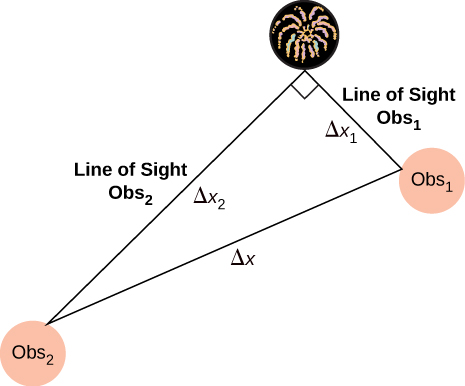$\begin{array}{}\\ \\ {T}_{\text{C}}=35\text{°}\text{C,}\phantom{\rule{0.5em}{0ex}}v=351.58\phantom{\rule{0.2em}{0ex}}\text{m/s}\hfill \\ \text{Δ}{x}_{1}=35.16\phantom{\rule{0.2em}{0ex}}\text{m,}\phantom{\rule{0.5em}{0ex}}\text{Δ}{x}_{2}=52.74\phantom{\rule{0.2em}{0ex}}\text{m}\hfill \\ \text{Δ}x=63.39\phantom{\rule{0.2em}{0ex}}\text{m}\hfill \end{array}$

The density of a sample of water is $\rho =998.00\phantom{\rule{0.2em}{0ex}}{\text{kg/m}}^{3}$ and the bulk modulus is $\beta =2.15\phantom{\rule{0.2em}{0ex}}\text{GPa}\text{.}$ What is the speed of sound through the sample?

Suppose a bat uses sound echoes to locate its insect prey, 3.00 m away. (See (Figure).) (a) Calculate the echo times for temperatures of $5.00\text{°}\text{C}$ and $35.0\text{°}\text{C}\text{.}$ (b) What percent uncertainty does this cause for the bat in locating the insect? (c) Discuss the significance of this uncertainty and whether it could cause difficulties for the bat. (In practice, the bat continues to use sound as it closes in, eliminating most of any difficulties imposed by this and other effects, such as motion of the prey.)

a. ${t}_{5.00\text{°}\text{C}}=0.0180\phantom{\rule{0.2em}{0ex}}\text{s},\phantom{\rule{0.5em}{0ex}}{t}_{35.0\text{°}\text{C}}=0.0171\phantom{\rule{0.2em}{0ex}}\text{s}$; b. $\text{% uncertainty}=5.00\text{%}$; c. This uncertainty could definitely cause difficulties for the bat, if it didn’t continue to use sound as it closed in on its prey. A 5% uncertainty could be the difference between catching the prey around the neck or around the chest, which means that it could miss grabbing its prey.